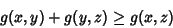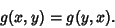## Metric

A Nonnegative functiondescribing the Distance'' between neighboring points for a given Set. A metric satisfies the Triangle Inequalityand is symmetric, soA Set possessing a metric is called a Metric Space. When viewed as a Tensor, the metric is called a Metric Tensor.

See also Cayley-Klein-Hilbert Metric, Distance, Fundamental Forms, Hyperbolic Metric, Metric Entropy, Metric Equivalence Problem, Metric Space, Metric Tensor, Part Metric, Riemannian Metric, Ultrametric

References

Gray, A. Metrics on Surfaces.'' Ch. 13 in Modern Differential Geometry of Curves and Surfaces. Boca Raton, FL: CRC Press, pp. 251-265, 1993.